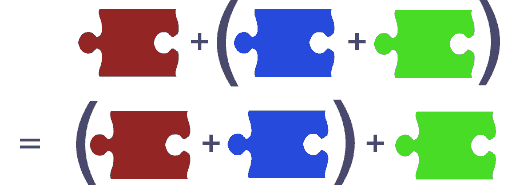# 函数式编程模式：半群Semigroup

函数式编程中一个重要概念是组合， 一个聚合体或复杂元素是由很多小部分组合而成。但是不是所有的组合形式是一样的，看看下面两个情况：

1. 拼图游戏的拼图组装
2. 组装一个宜家家具### 定义

`a ⊕ (b ⊕ c) = (a ⊕ b) ⊕ c`

### 案例

`a + (b + c) = (a + b) + c`
`a * (b * c) = (a * b) * c`
`max(a, max(b, c)) = max(max(a, b), c)`
`min(a, min(b, c)) = min(min(a, b), c) `

`a ++ (b ++ c) = (a ++ b) ++ c`

[1, 2] ++ [3, 4, 5] 等同于 [1, 2, 3, 4, 5]

`a && (b && c) = (a && b) && c`
`a || (b || c) = (a || b) || c `

`f ∘ (g ∘ h) = (f ∘ g) ∘ h`

`{ A: 2, B: 4, C: 3, D: 1 }`

`addFrequencies({ A: 2, B: 1, C: 3 }, { B: 3, C: 1, D: 5 })  >> { A: 2, B: 4, C: 4, D: 5 }`

`function compareNumbers(i, j) {      return i - j;  }`

```function composeComparators(c, d) {
return (x, y) => {
const comparisonResult = d(x, y);
if (comparisonResult === EQUAL) {
return c(x, y);
}
return comparisonResult;
};
} ```

`x +） y = y`

Javascript专题